## Overview

• Important high-level plotting functions
• `plot`: generic x-y plotting
• `barplot`: bar plots
• `boxplot`: box-and-whisker plot
• `hist`: histograms
• `pie`: pie charts
• `dotchart`: cleveland dot plots
• `image, heatmap, contour, persp`: functions to generate image-like plots
• `qqnorm, qqline, qqplot`: distribution comparison plots
• `pairs, coplot`: display of multivariant data
• Help on these functions
• `?myfct`</span></span>
• `?plot`</span></span>
• `?par`</span></span>

## Preferred Input Data Objects

• Matrices and data frames
• Vectors
• Named vectors

## Scatter Plots

### Basic scatter plots

Sample data set for subsequent plots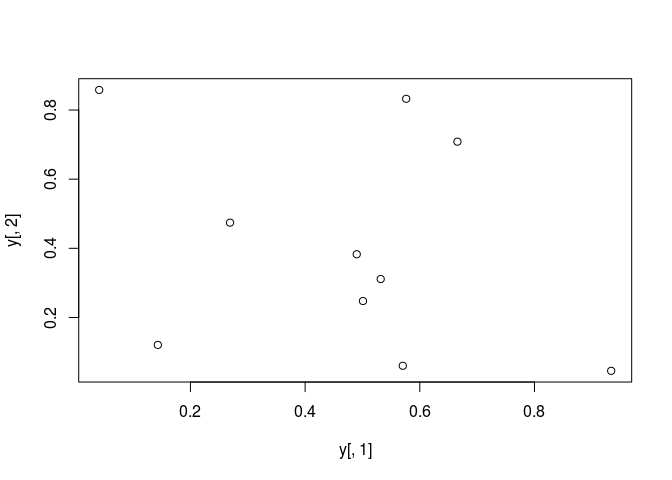### All pairs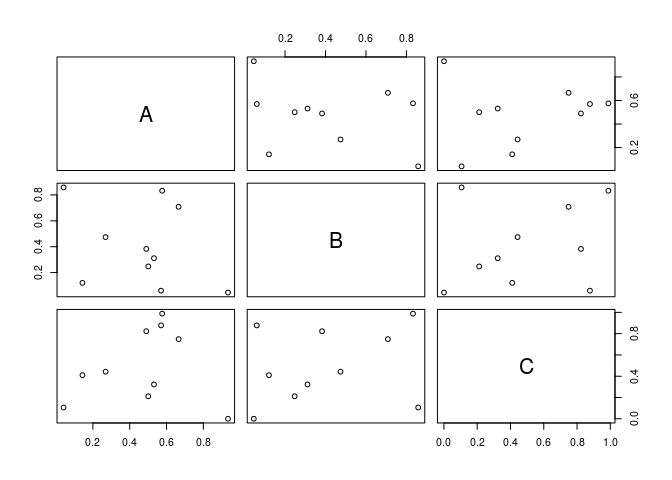### Plot labels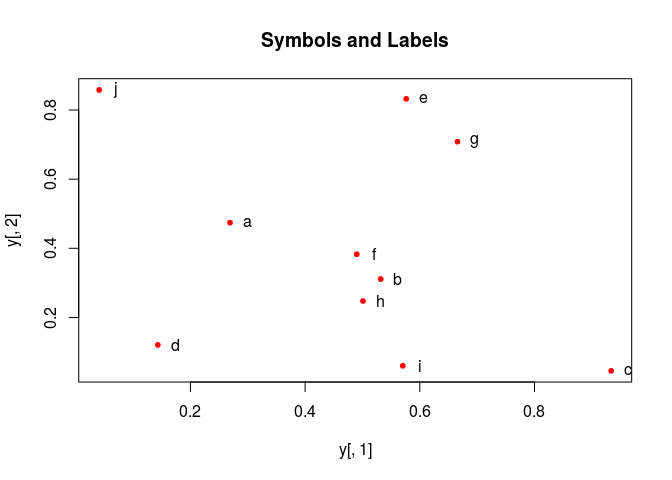### More examples

Print instead of symbols the row names

Usage of important plotting parameters

Important arguments} - `mar`: specifies the margin sizes around the plotting area in order: `c(bottom, left, top, right)` - `col`: color of symbols - `pch`: type of symbols, samples: `example(points)` - `lwd`: size of symbols - `cex.*`: control font sizes - For details see `?par`

Add a regression line to a plot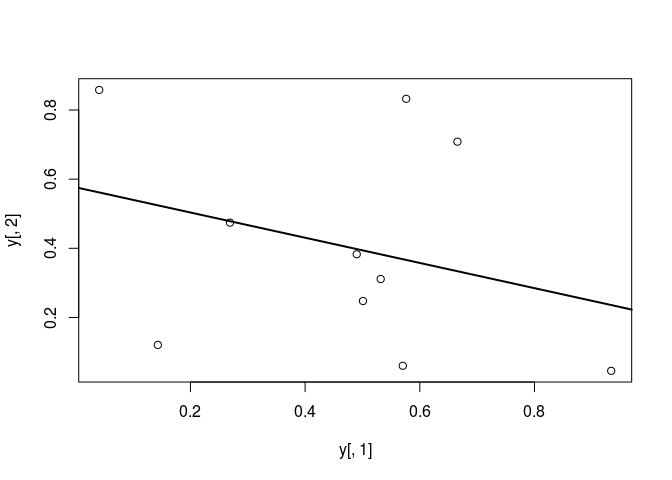Same plot as above, but on log scale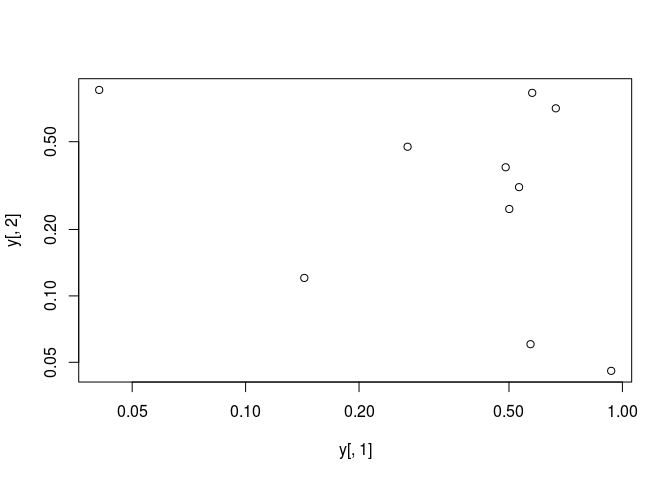Add a mathematical expression to a plot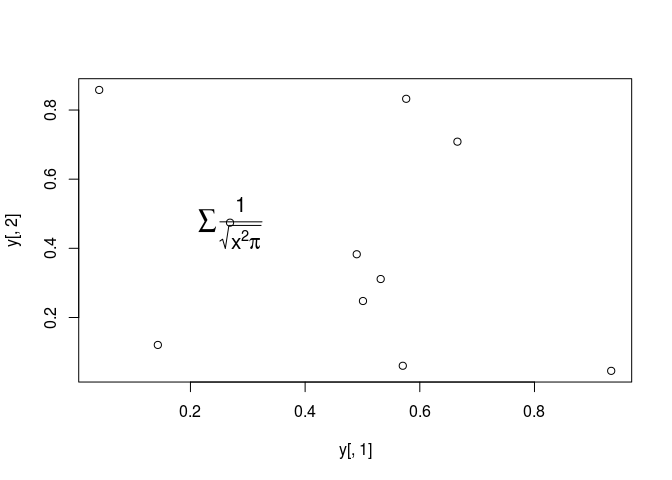### Exercise 1

• Task 1: Generate scatter plot for first two columns in `iris` data frame and color dots by its `Species` column.
• Task 2: Use the `xlim/ylim` arguments to set limits on the x- and y-axes so that all data points are restricted to the left bottom quadrant of the plot.

Structure of iris data set:

## Line Plots

### Single Data Set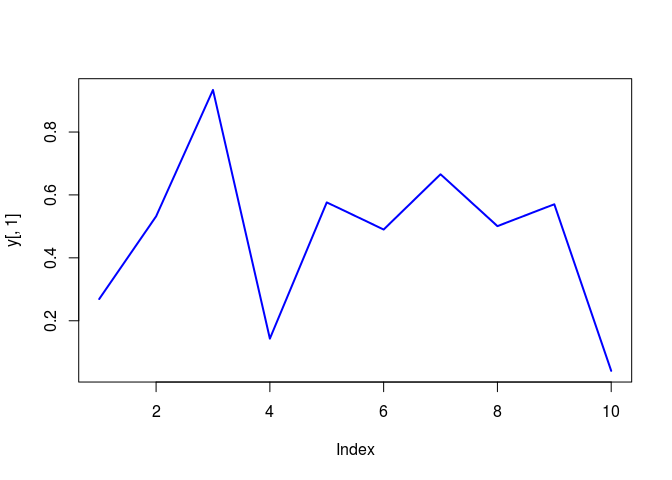### Many Data Sets

Plots line graph for all columns in data frame `y`. The `split.screen` function is used in this example in a for loop to overlay several line graphs in the same plot.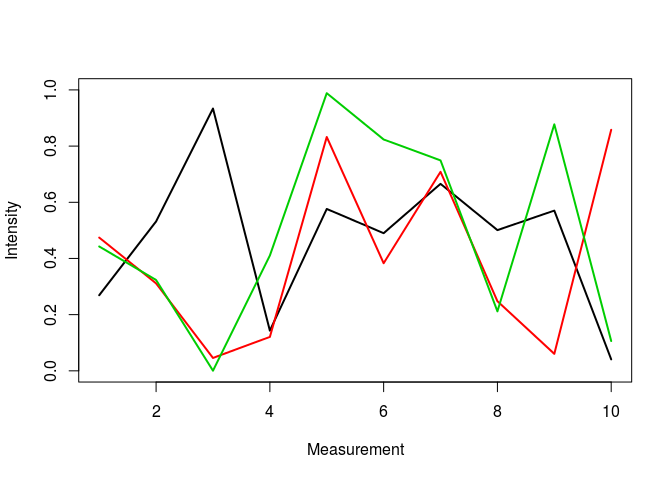## Bar Plots

### Basics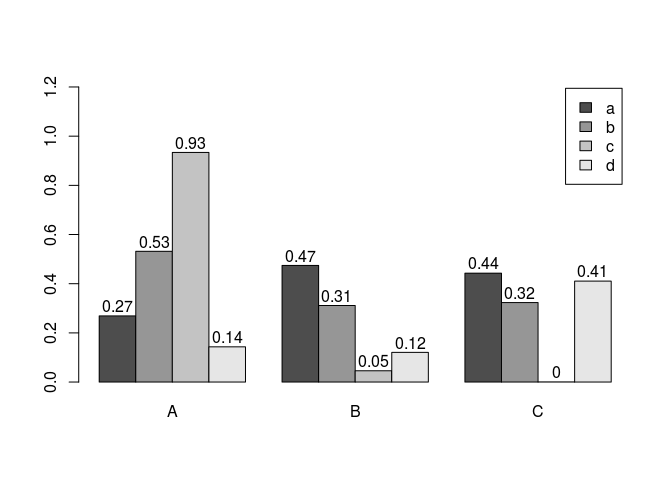### Error bars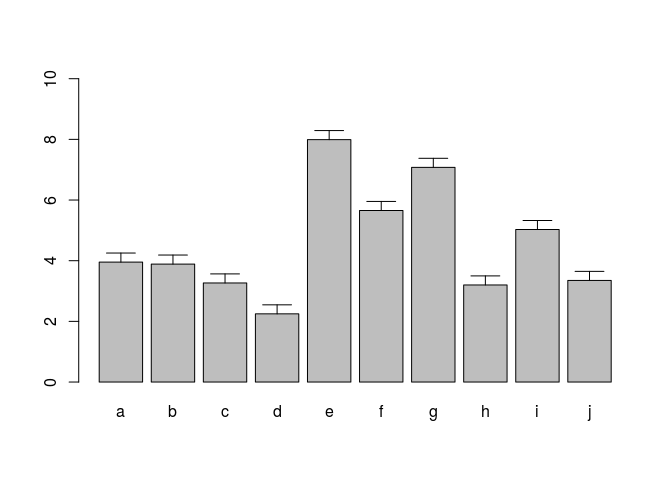### Mirrored bar plot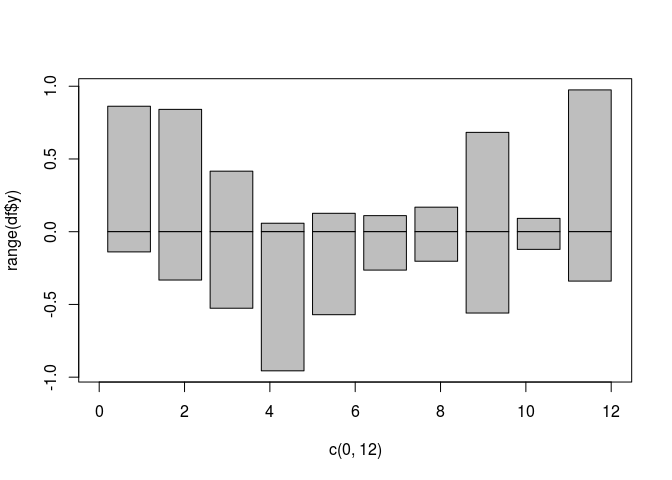## Histograms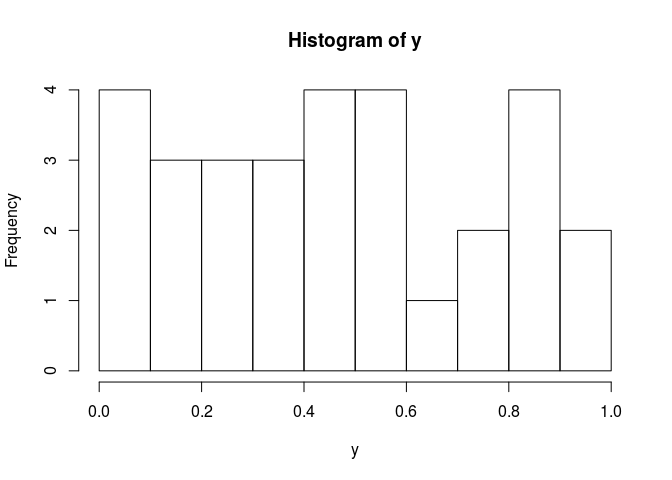## Density Plots}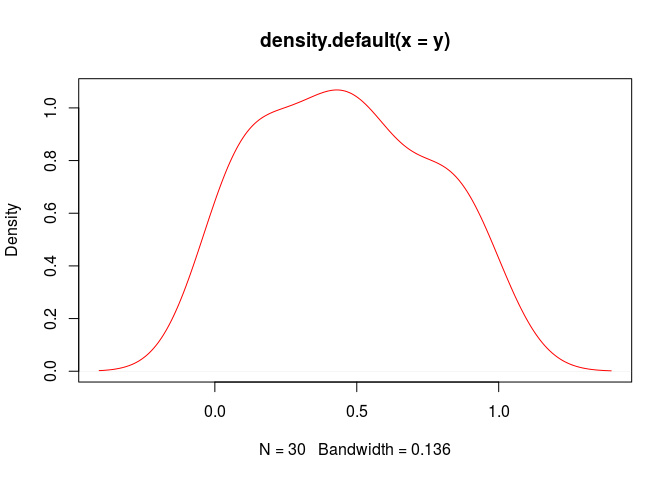## Pie Charts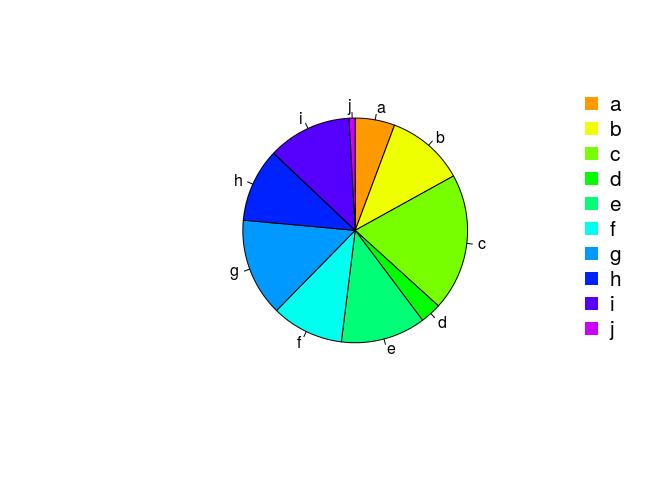## Color Selection Utilities

Default color palette and how to change it

The `gray` function allows to select any type of gray shades by providing values from 0 to 1

Color gradients with `colorpanel` function from `gplots` library

Much more on colors in R see Earl Glynn’s color chart

## Arranging Several Plots on Single Page

With `par(mfrow=c(nrow,ncol))` one can define how several plots are arranged next to each other.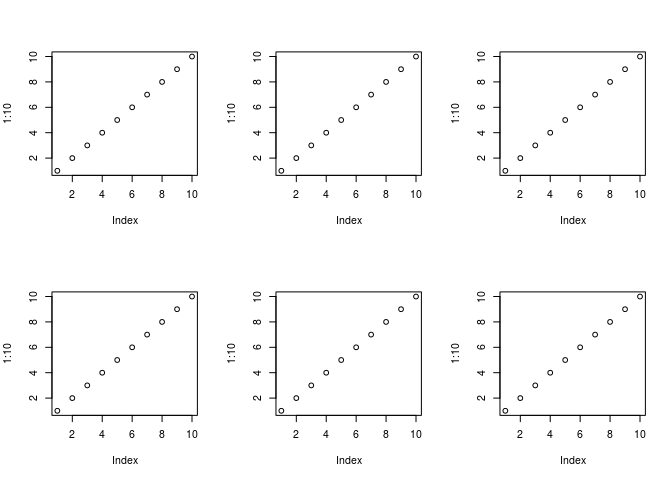### Arranging Plots with Variable Width

The `layout` function allows to divide the plotting device into variable numbers of rows and columns with the column-widths and the row-heights specified in the respective arguments.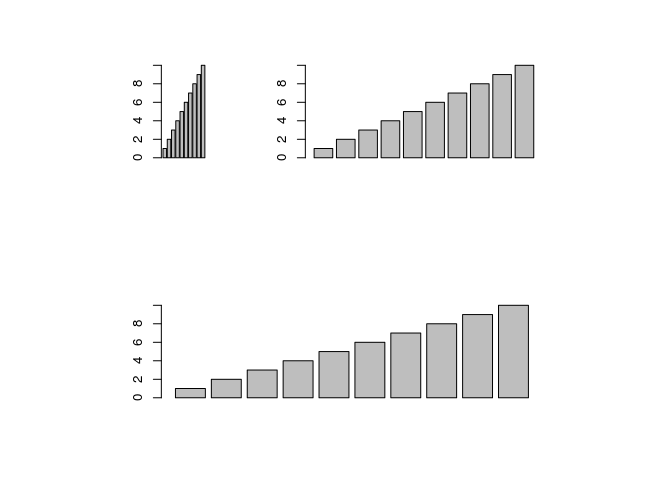## Saving Graphics to Files

After the `pdf()` command all graphs are redirected to file `test.pdf`. Works for all common formats similarly: jpeg, png, ps, tiff, …

Generates Scalable Vector Graphics (SVG) files that can be edited in vector graphics programs, such as InkScape.

### Exercise 2

Bar plots

• Task 1: Calculate the mean values for the \Rfunction{Species} components of the first four columns in the \Rfunction{iris} data set. Organize the results in a matrix where the row names are the unique values from the \Rfunction{iris Species} column and the column names are the same as in the first four \Rfunction{iris} columns.
• Task 2: Generate two bar plots: one with stacked bars and one with horizontally arranged bars.

Structure of iris data set: